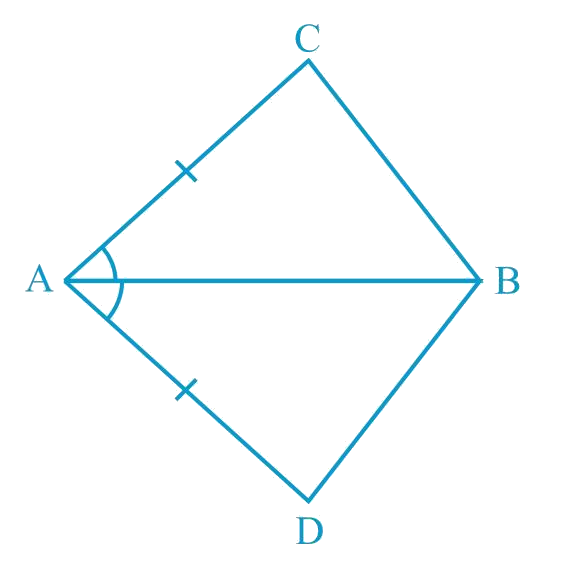# Ex.7.1 Q1 Triangles Solution - NCERT Maths Class 9

## Question

In quadrilateral $$ACBD, AC = AD$$ and $$AB$$ bisects $$\angle A$$ (See the given figure). Show that \begin{align} \Delta ABC \cong \Delta ABD \end{align}. What can you say about $$BC$$ and $$BD$$?Video Solution
Triangles
Ex 7.1 | Question 1

## Text Solution

What is Known?

$$AC = AD$$ and $$AB$$ bisects

To prove:

$$\Delta {\text{ABC}} \cong \Delta {\text{ABD}}$$ and, what can be said about $$BC$$ and $$BD.$$

Reasoning:

We can show two sides and included angle of are equals to corresponding sides and included angle of by using SAS congruency criterion both triangles will be congruent and by CPCT, BC and BD will be equal.Steps:

In $$\Delta ABC$$ and $$\Delta ABD$$,

\begin{align} AC &= AD \text {(Given)}\\\\\Delta CAB &=\Delta DAB\\&(AB \text { bisects } \angle A)\\\\AB &= AB\\ &\text {(Common)}\\ \\ \therefore \Delta ABC &\cong \Delta ABD\\ &\text {(By congruence rule)}\\ \\\therefore BC&=BD\\&\left(\begin{array}{I} \text{By corresponding parts}\, \\ \text{of congruent triangles} \\ \end{array}\!\right)\end{align}

Therefore, $$BC$$ and $$BD$$ are of equal lengths.

Video Solution
Triangles
Ex 7.1 | Question 1
Learn from the best math teachers and top your exams

• Live one on one classroom and doubt clearing
• Practice worksheets in and after class for conceptual clarity
• Personalized curriculum to keep up with school